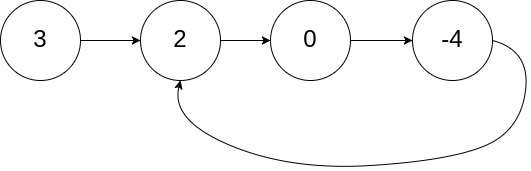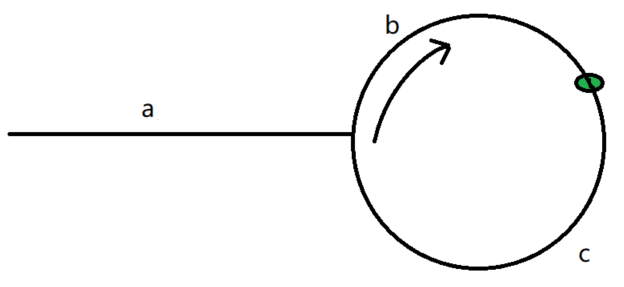# 精选算法面试 - 链表（判断环）## 二.示例

### 2.1 判断链表是否有环public boolean hasCycle(ListNode head) {        ListNode node = head;        boolean result = false;        if(node == null){            return result;        }        //快指针        ListNode next = head.next;        if(next == null){            return result;        }        while (next != null && next.next != null){            //快指针与慢指针相遇，证明有环            if(next == node){                result = true;                break;            }            next = next.next.next;            //慢指针            node = node.next;        }        return result;    }

### 2.2 环形链路入口结点public ListNode detectCycle(ListNode head) {        ListNode pos =head;        //通过集合判断是否存在环        Set<ListNode> visited  = new HashSet<>();        while (pos != null){            if(visited.contains(pos)){                return pos;            }else{                visited.add(pos);            }            pos = pos.next;        }        return null;}fast 指针走过距离 a+n(b+c)+b=a+(n+1)b+nca+n(b+c)+b=a+(n+1)b+nc。

a+(n+1)b+nc=2(a+b)⟹a=c+(n−1)(b+c)

• 快针走的是慢针的两倍。

• 慢针走过的路，快针走过一遍。

• 快针走过的剩余路程，也就是和慢针走过的全部路程相等。(a+b = c+b)

• 刨去快针追赶慢针的半圈(b)，剩余路程即为所求入环距离(a=c)

public ListNode detectCycle(ListNode head) {        ListNode node = head;        if(node == null){            return null;        }        ListNode next = head;               while (next != null){            node = node.next;            //快指针            if(next.next != null){                next = next.next.next;             }else{                return null;            }            //快指针与慢指针相遇            if(next == node){                //头结点                ListNode p = head;                //从头结点出发查找迭代到相遇点位置                while(p != node){                    p = p.next;                    node = node.next;                }                return p;            }          }        return null;    }## 评论